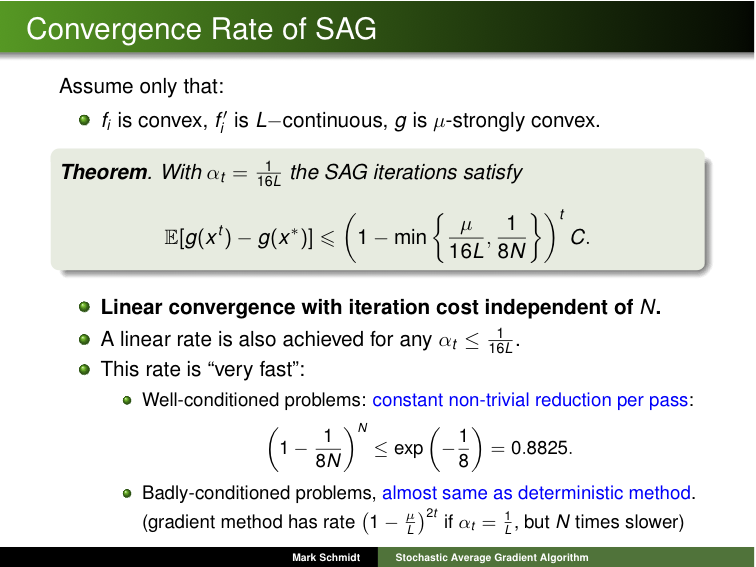# SAG: Minimizing Finite Sums with the Stochastic Average Gradient

By Mark Schmidt (2013)
Last updated 30 Sep 2013.

## Summary

The SAG code contains C implementations (via Matlab mex files) of the stochastic average gradient (SAG) method, as well as several related methods, for the problem of L2-regularized logistic regression with a finite training set.

The SAG method is described in the following papers:

Clicking on the image below opens the slides of a talk giving the motivation for the new algorithm, a summary of theoretical results, and some experimental results:The specific methods available in the package are:
• SGD: The stochastic gradient method with (user-supplied) step-sizes, (optional) projection step, and (optional) (weighted-)averaging.
• ASGD: A variant of the above code that supports less features, but efficiently implements uniform averaging on sparse data sets.
• PCD: A basic primal coordinate descent method with step sizes set according to the (user-supplied) Lipschitz constants.
• DCA: A dual coordinate ascent method with a Newton-based line-search.
• SAG: The stochastic average gradient method with a (user-supplied) constant step size.
• SAGlineSearch: The stochastic average gradient method with the line-search described in the paper.
• SAG_LipschitzLS: The stochastic average gradient method with the line-search and adaptive non-uniform sampling strategy described in the paper.
With the exception of the last method which chooses the data point to compute the gradient on adaptively, all methods require the sequence of data points to process to be provided as an input parameter. With the exception of the first method when averaging the iterations, all of the codes avoid full-vector operations when the input is sparse. For each of the above, there are two versions: (1) a normal version that only uses basic C commands and (2) a variant that replaces some of these operations by BLAS commands. For some problems and architectures, the BLAS variants of the code could up to almost 4 times faster on dense data sets. The BLAS variants have the ".c" in their file names replaced by "_BLAS.c" (they do not seem to compile on the most recent versions of MatLab).

Note that for the methods that use a line-search, the line-search has been implemented so that it does not depend on the number of examples nor the number of variables.

Once this is done, type the following in Matlab:

```>> cd SAG                  % Change to the unzipped directory
>> mexAll                  % Compile mex files (not necessary on all systems)
>> example_SAG             % Run all of the basic methods on a sparse data set
>> example_SAG2             % Run all of the basic methods (plus non-uniform sampling) on a dense data set
```
If you get them compiled, the script example_SAG_BLAS shows how to run the variants that use BLAS on the dense dataset.

## Extensions

It should be straightforward to modify these C codes to compute parameters in other L2-regularized linearly-parameterized models, by changing the value of the 'sig' variable that represents the derivative of the loss. For example, we modified the SGD code to solve for support vector machines in this report:
To modify the methods relying on a line-search, you would also have to modify the values of 'fi' and 'fi_new' in the C code to compute the alternate loss value. To consider other types of regularizers or loss functions, more changes would need to be made but the overall structure of the method could be the same.

For example, we have made a variant of this code that applies to conditional random fields (CRFs) available here.

Mark Schmidt > Software > SAG